# 为什么说神经网络可以逼近任意函数？• 收集关键数据（大量人口的身高/体重/年龄，已经对应的实际服装尺寸）。
• 训练模型来实现输入-输出的映射逼近。
• 对未知数据进行预测来验证模型。

## 人工神经网络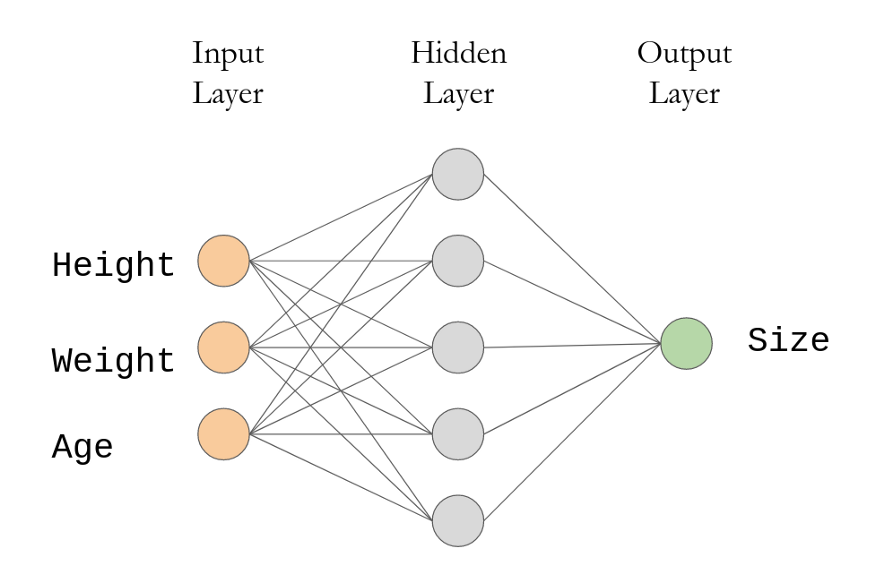## 非线性关系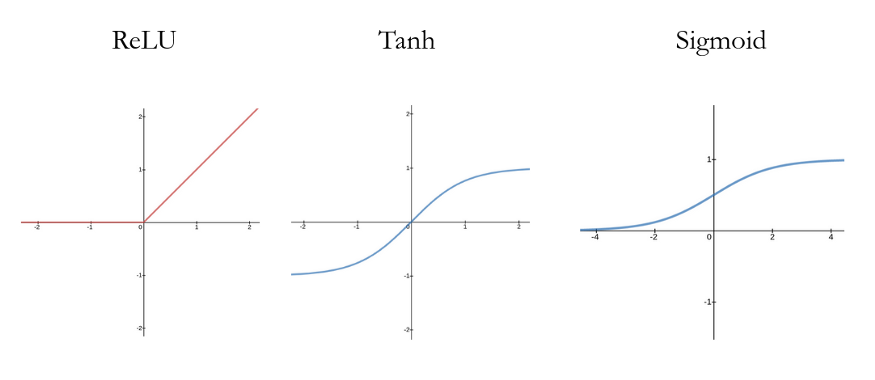ReLU是一个简单的分段线性函数-计算消耗小。另外两个都涉及到指数运算，因此计算成本更高

## 案例一：任意散点曲线拟合

import torch
import plotly.graph_objects as go
import numpy as np

# Batch Size, Input Neurons, Hidden Neurons, Output Neurons
N, D_in, H, D_out = 16, 1, 1024, 1

# Create random Tensors to hold inputs and outputs
x = torch.randn(N, D_in)
y = torch.randn(N, D_out)

# Use the nn package to define our model
# Linear (Input -> Hidden), ReLU (Non-linearity), Linear (Hidden-> Output)
model = torch.nn.Sequential(
torch.nn.Linear(D_in, H),
torch.nn.ReLU(),
torch.nn.Linear(H, D_out),
)

# Define the loss function: Mean Squared Error
# The sum of the squares of the differences between prediction and ground truth
loss_fn = torch.nn.MSELoss(reduction='sum')

# The optimizer does a lot of the work of actually calculating gradients and
# applying backpropagation through the network to update weights
learning_rate = 1e-4

# Perform 30000 training steps
for t in range(30000):
# Forward pass: compute predicted y by passing x to the model.
y_pred = model(x)

# Compute loss and print it periodically
loss = loss_fn(y_pred, y)
if t % 100 == 0:
print(t, loss.item())

# Update the network weights using gradient of the loss
loss.backward()
optimizer.step()

# Draw the original random points as a scatter plot
fig = go.Figure()

# Generate predictions for evenly spaced x-values between minx and maxx
minx = min(list(x.numpy()))
maxx = max(list(x.numpy()))
c = torch.from_numpy(np.linspace(minx, maxx, num=640)).reshape(-1, 1).float()
d = model(c)

# Draw the predicted functions as a line graph
fig.show()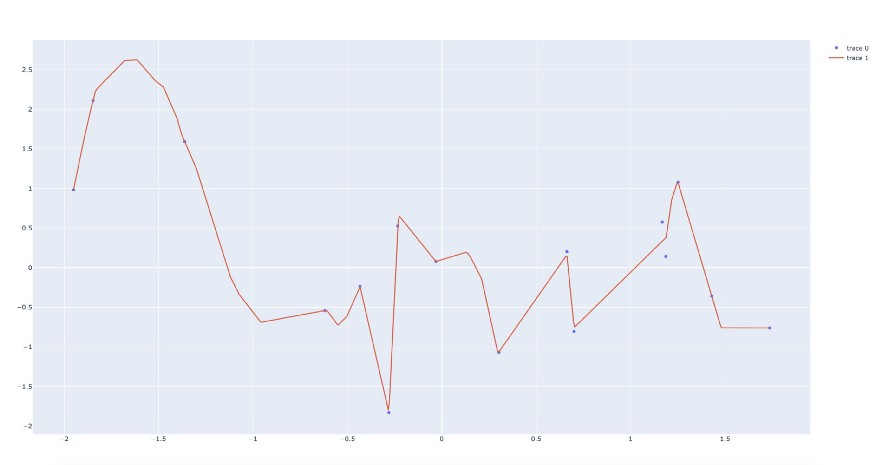## 案例二：二值分类

import torch
import plotly.express as px
import pandas as pd

# Batch Size, Input Neurons, Hidden Neurons, Output Neurons
N, D_in, H, D_out = 128, 2, 1024, 1

# Create random Tensors to hold inputs and outputs
x = torch.rand(N, D_in)
y = torch.randint(0, 2, (N, D_out))

# Plot randomly generated points and color by label
df = pd.DataFrame({"x": x[:, 0].flatten(), "y": x[:, 1].flatten(), "class": y.flatten()})
fig = px.scatter(df, x="x", y="y", color="class", color_continuous_scale="tealrose")
fig.show()

# define model: Linear (Input->Hidden), ReLU, Linear (Hidden->Output), Sigmoid
model = torch.nn.Sequential(
torch.nn.Linear(D_in, H),
torch.nn.ReLU(),
torch.nn.Linear(H, D_out),
torch.nn.Sigmoid()
)

# define loss function: Binary Cross Entropy Loss (good for binary classification tasks)
loss_fn = torch.nn.BCELoss()

learning_rate = 0.002

# Store losses over time
ts, losses = ([], [])

# run training steps
for t in range(60000):
y_pred = model(x)

loss = loss_fn(y_pred.float(), y.float())
if t % 100 == 0:
ts.append(t)
losses.append(loss.data.numpy())

loss.backward()
optimizer.step()

# generate a bunch of random points to cover the sample space, then call model
c = torch.rand(32000, D_in)
d = model(c)

# store random data and predicted classifications in a DataFrame and plot with Plotly Express
df2 = pd.DataFrame({"x": c[:, 0].flatten(),
"y": c[:, 1].flatten(),
"class": d.flatten().detach().numpy()})
fig2 = px.scatter(df2, x="x", y="y", color="class", color_continuous_scale="tealrose")
fig2.show()

# plot the loss as a function of training step
fig3 = px.scatter(x=ts, y=losses)
fig3.show()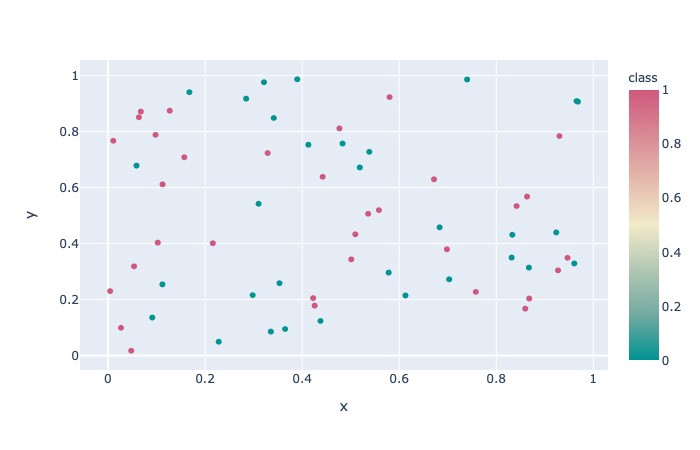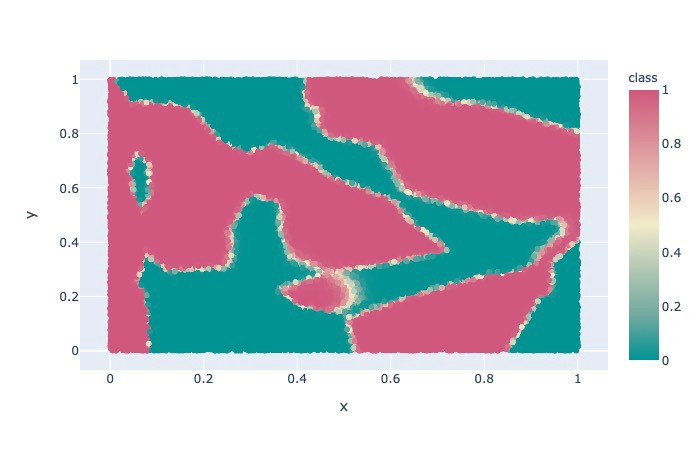## 结语

deephub翻译组 Oliver Lee

10-173152

#### ReLU深度网络能逼近任意函数的原因03-156753

#### 浅析神经网络为什么能够无限逼近任意连续函数？

09-1852

#### 【神经网络和深度学习】笔记 - 第四章 神经网络可以实现任意函数的直观解释【转】...

04-033220

#### 为什么神经网络能以任意精度拟合任意复杂度的函数？——转自简书

06-102010

#### DeepLearning学习笔记——万能近似定理

09-083201

#### 神经网络的激活函数为什么要使用非线性函数？

01-114520

#### 利用神经网络逼近sin(x)函数

12-09319

#### 为什么神经网络需要非线性激活函数©️2020 CSDN 皮肤主题: 技术工厂 设计师: CSDN官方博客点击重新获取扫码支付1.余额是钱包充值的虚拟货币，按照1:1的比例进行支付金额的抵扣。
2.余额无法直接购买下载，可以购买VIP、C币套餐、付费专栏及课程。余额充值